# Bias Removal¶

Climate models can have biases relative to different verification datasets. Commonly, biases are removed by postprocessing before verification of forecasting skill. climpred provides convenience functions to do so.

The select from following methods by specifying how in HindcastEnsemble.remove_bias:

• how="additive_mean": correcting the mean forecast additively

• how="multiplicative_mean": correcting the mean forecast multiplicatively

• how="multiplicative_std": correcting the standard deviation multiplicatively

Wrapped from the github package bias_correction:

• how="modified_quantile": Reference

• how="basic_quantile": Reference

• how="gamma_mapping": Reference

• how="normal_mapping": Reference

Wrapped from xlim.sdba:

:

# linting

:

import climpred
import xarray as xr
import matplotlib.pyplot as plt
from climpred import HindcastEnsemble

:

init = climpred.tutorial.load_dataset("NMME_hindcast_Nino34_sst")
v = "sst"

:

hindcast = HindcastEnsemble(init.sel(model="GFDL-CM2p5-FLOR-A06")).add_observations(obs)
hindcast.plot()

/Users/aaron.spring/Coding/climpred/climpred/checks.py:256: UserWarning: Did not find dimension "init", but renamed dimension S with CF-complying standard_name "forecast_reference_time" to init.
warnings.warn(
/Users/aaron.spring/Coding/climpred/climpred/checks.py:256: UserWarning: Did not find dimension "member", but renamed dimension M with CF-complying standard_name "realization" to member.
warnings.warn(
/Users/aaron.spring/Coding/climpred/climpred/checks.py:256: UserWarning: Did not find dimension "lead", but renamed dimension L with CF-complying standard_name "forecast_period" to lead.
warnings.warn(

:

<AxesSubplot:xlabel='time', ylabel='Sea Surface Temperature\n[Celsius_scale]'>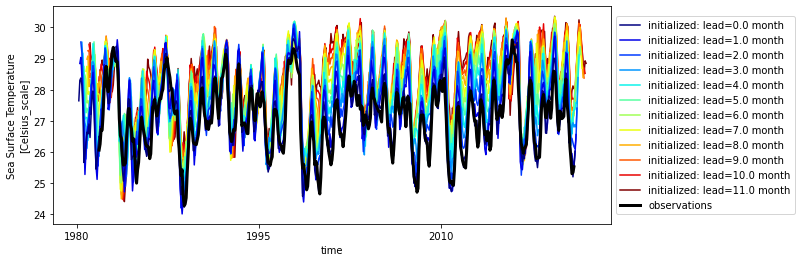The warming of the observations is similar to initialized.

Typically, bias depends on lead-time and therefore should therefore also be removed depending on lead.

:

bias = hindcast.verify(
)

bias[v].plot()

:

<matplotlib.collections.QuadMesh at 0x14e529ac0>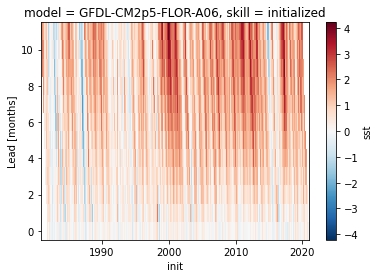:

# group bias by seasonality
seasonality = climpred.options.OPTIONS["seasonality"]
seasonality

:

'month'

:

bias.groupby(f"init.{seasonality}").mean()[v].plot()

:

<matplotlib.collections.QuadMesh at 0x14f097e80>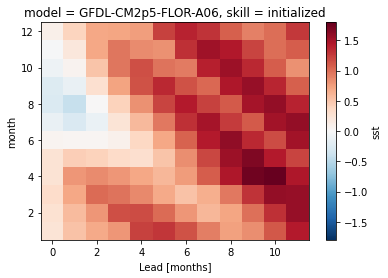An initial warm bias develops into a cold bias, especially in winter.

## train_test_split¶

Risbey et al. 2021 demonstrate how important a clean separation of a train and a test period is for bias reduction.

Implemented train_test_splits in climpred:

• unfair: completely overlapping train and test (climpred default)

• unfair-cv: overlapping train and test except for current init, which is left out (set cv='LOO')

• fair: no overlap between train and test (recommended)

:

metric_kwargs = dict(
metric="rmse", alignment="same_verifs", dim="init", comparison="e2o", skipna=True
)

:

# fair calculates bias for train_time/train_init and drops these data from hindcast
hindcast.remove_bias(
alignment=metric_kwargs["alignment"],
train_test_split="fair",
train_time=slice("1982", "1998"),
).plot()

:

<AxesSubplot:xlabel='time', ylabel='Sea Surface Temperature\n[Celsius_scale]'>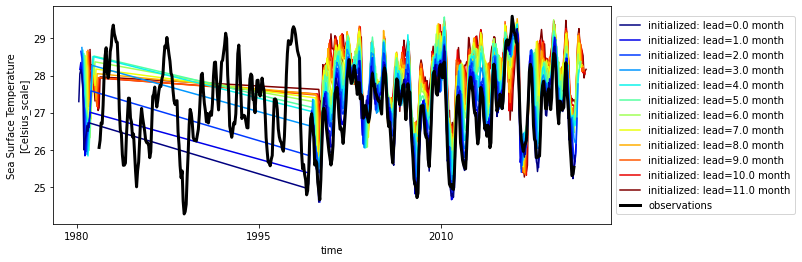:

hindcast.verify(**metric_kwargs)[v].plot(label="no bias correction")
train_test_split = ["unfair", "unfair-cv", "fair"]

hindcast.remove_bias(
).verify(**metric_kwargs)[v].plot(label="unfair")

hindcast.remove_bias(
alignment=metric_kwargs["alignment"],
train_test_split="unfair-cv",
cv="LOO",
).verify(**metric_kwargs)[v].plot(label="unfair-cv")

hindcast.remove_bias(
alignment=metric_kwargs["alignment"],
train_test_split="fair",
train_time=slice("1982", "1998"),
).verify(**metric_kwargs)[v].plot(label="fair")

plt.legend()
plt.title(
f"NMME {hindcast.coords['model'].values} Nino3.4 SST {metric_kwargs['metric'].upper()}"
)

:

Text(0.5, 1.0, 'NMME GFDL-CM2p5-FLOR-A06 Nino3.4 SST RMSE')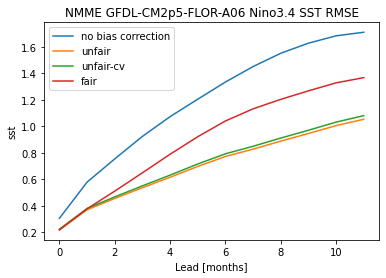# Comparison of methods how¶

:

methods = [
# "multiplicative_std",
"DetrendedQuantileMapping",
"EmpiricalQuantileMapping",
# "PrincipalComponents",
# "LOCI",
"QuantileDeltaMapping",
"Scaling",
"modified_quantile",
"basic_quantile",
# "gamma_mapping",
# "normal_mapping",
]

:

import warnings

warnings.simplefilter("ignore")

:

# xclim.sdba requires pint units
hindcast._datasets["initialized"][v].attrs["units"] = "C"
hindcast._datasets["observations"][v].attrs["units"] = "C"

:

hindcast.sel(init=slice("1983", "2018")).verify(**metric_kwargs)[v].plot(label="raw")
for method in methods:
hindcast.remove_bias(
how=method, alignment=metric_kwargs["alignment"], train_test_split="unfair"
).verify(**metric_kwargs)[v].plot(label=method)
plt.legend()

:

<matplotlib.legend.Legend at 0x14ed73850>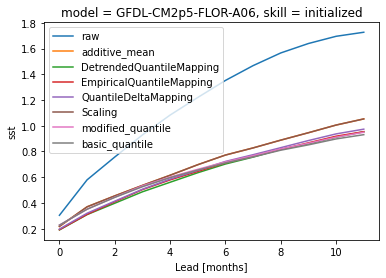[ ]: